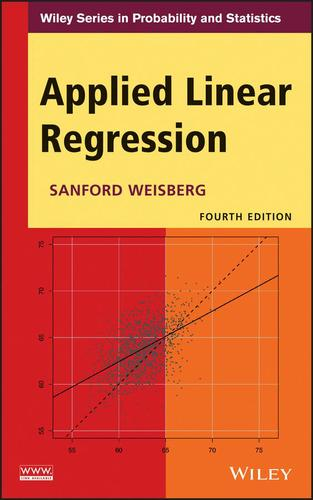# Applied Linear Regression

4th Edition

Sanford Weisberg
eISBN-13: 9781118789551## eBook Features

or
Rent or Buy from \$ 116.00 USD
Note: We do not guarantee supplemental material with textbooks (e.g. CD's, Music, DVD's, Access Code, or Lab Manuals)

Praise for the Third Edition

"...this is an excellent book which could easily be used as a course text..."
—International Statistical Institute

The Fourth Edition of Applied Linear Regression provides a thorough update of the basic theory and methodology of linear regression modeling. Demonstrating the practical applications of linear regression analysis techniques, the Fourth Edition uses interesting, real-world exercises and examples.

Stressing central concepts such as model building, understanding parameters, assessing fit and reliability, and drawing conclusions, the new edition illustrates how to develop estimation, confidence, and testing procedures primarily through the use of least squares regression. While maintaining the accessible appeal of each previous edition,Applied Linear Regression, Fourth Edition features:

Graphical methods stressed in the initial exploratory phase, analysis phase, and summarization phase of an analysis In-depth coverage of parameter estimates in both simple and complex models, transformations, and regression diagnostics Newly added material on topics including testing, ANOVA, and variance assumptions Updated methodology, such as bootstrapping, cross-validation binomial and Poisson regression, and modern model selection methods

Applied Linear Regression, Fourth Edition is an excellent textbook for upper-undergraduate and graduate-level students, as well as an appropriate reference guide for practitioners and applied statisticians in engineering, business administration, economics, and the social sciences.

Sold By Wiley 9781118386088, 9781118789551, 9781118386088 eng 370 4th